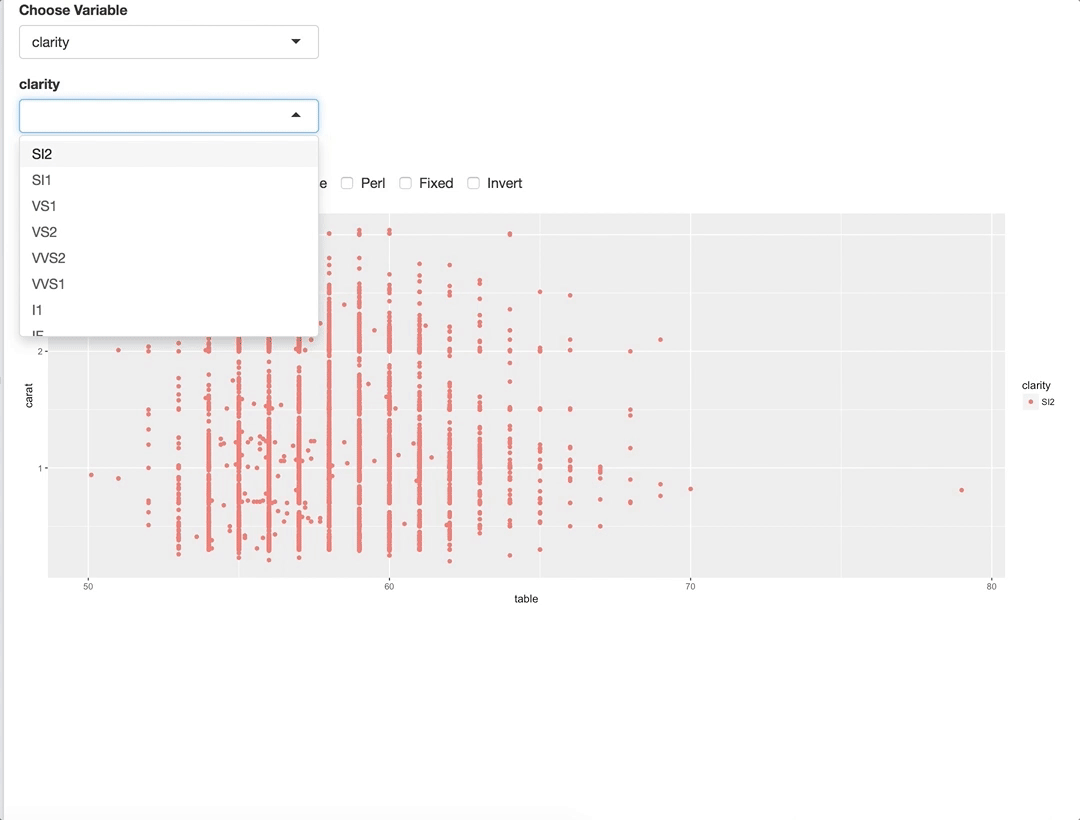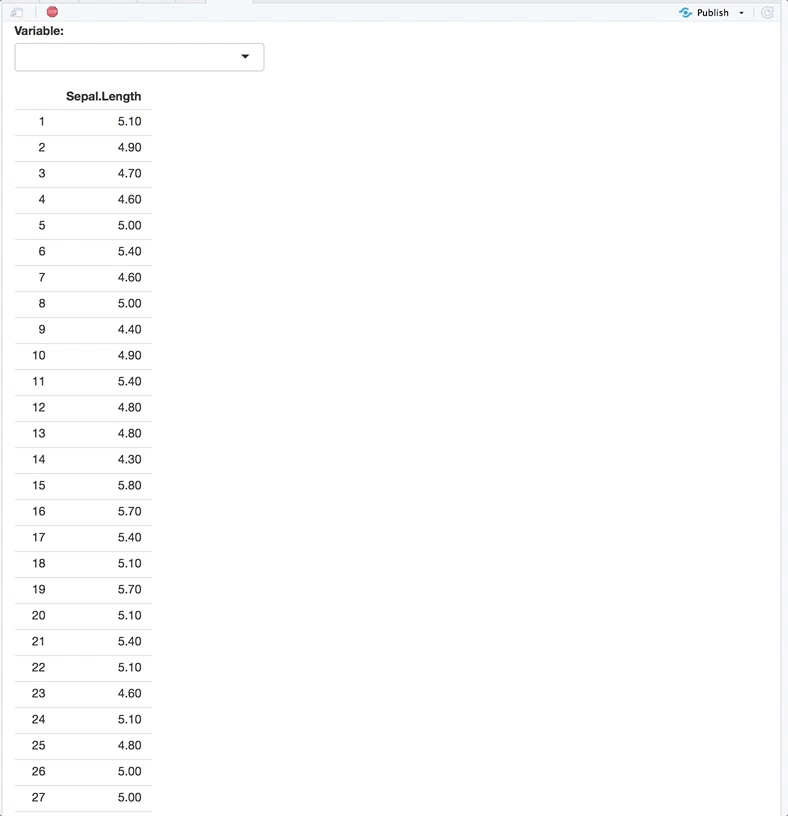Want to share your content on R-bloggers? click here if you have a blog, or here if you don't.

regexSelect is a small package that uses Shiny modules to solve a problem in Shiny selectize objects – regular expression (regex) searching. You can quickly filter the values in the selectize object, while being able to add that new regex query to the selectize list.

This is great for long lists, since you can return multiple item simultaneously without needing to endlessly click items in a list!

## Install

```install.packages('regexSelect')
#devtools::install_github('yonicd/regexSelect')
```

Below are two examples of using regular expressions to quickly populate multiple items in a `ggplot` and a `datatable`.

## regexSelect with PlotsThe shiny module works with two main functions:

```# server side:
callModule(module=regexSelect, id='myId', reactive(<selectizeInput Choices>))

# ui side:
regexSelectUI(id = "myId", label = 'myLabel', choices = <selectizeInput Choices>)
```

regexSelect comes with controls placed in a group checkbox below the selectize object. When calling regexSelect you can show or hide the checkbox controls (hidden by default), as to make it look like a normal selectize object, and save valuable UI real estate.

While the shiny app is running regexSelect properties can be manipulated through the checkbox controls giving greater flexibilty to:

• Toggle regexSelect to work as a standard selectize object.
• Retain the regex search as a new value the selectize object.
• Change arguments that are passed to base::grep : `ignore.case`, `perl`, `fixed`, `invert`.
```library(shiny)
library(ggplot2)

ui <- fluidPage(

selectInput('var',
'Choose Variable',
choices = names(diamonds)[sapply(diamonds,function(x){
inherits(x,c('character','factor')))]
},
selected = 'clarity'),

uiOutput('regexchoose'),

plotOutput("data")

)

server <- function(input, output, session) {

output\$regexchoose<-shiny::renderUI({

regexSelectUI(id = "a",
label = input\$var,
choices = unique(diamonds[[input\$var]]),
checkbox.show = TRUE)
})

observeEvent(input\$var,{

curr_cols <- callModule(module = regexSelect,
id = "a",
shiny::reactive(unique(diamonds[[input\$var]]))
)

observeEvent(curr_cols(),{

cols_now <- curr_cols()

output\$data <- shiny::renderPlot({

ggplot(diamonds[diamonds[[input\$var]]%in%cols_now,],
aes_string(x='table',y='carat',colour=input\$var))+
geom_point()

})
})
})

}

shinyApp(ui, server)
```

## regexSelect with Tables```ui <- shiny::fluidPage(

regexSelectUI(id = "a",
label = "Variable:",
choices = names(iris)
),

shiny::tableOutput("data")

)

server <- function(input, output, session) {

curr_cols <- callModule(module = regexSelect,
id = "a",
shiny::reactive(names(iris))
)

observeEvent(curr_cols(),{

cols_now <- curr_cols()

if( length(cols_now)==0 )
cols_now <- names(iris)

output\$data <- shiny::renderTable({

iris[,cols_now , drop = FALSE]

}, rownames = TRUE)

})
}

shiny::shinyApp(ui, server)
```